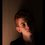# Indian INMO 2018 - problems

$\bf 1. \rm$ Let $ABC$ be a non-equilateral triangle with integer sides. Let $D$ and $E$ be respectively the mid-points $BC$ and $CA$; let $G$ be the centroid of triangle $ABC$. Suppose $D,C,E,G$ are concylic. Find the least possible perimeter of triangle $ABC$.

$\bf 2. \rm$ For any natural number $n$, consider a $1\times n$ rectangular board made up of $n$ unit squares. This is covered by three types of tiles: $1\times 1$ $\color{#D61F06} \text{red} \color{#333333}$ tile, $1\times 1$ $\color{#20A900} \text{green} \color{#333333}$ tile and $1\times 2$ $\color{#3D99F6} \text{blue} \color{#333333}$ domino. (For example, we can have $5$ types of tiling when $n=2$: red-red,red-green, green-red, green-green and blue.) Let $t_n$ denote the number of ways of covering $1\times n$ rectangular board by these three types of tiles. Prove that $t_n$ divides $t_{2n+1}$.

$\bf 3. \rm$ Let $\Gamma_1$ and $\Gamma_2$ be two circles with respective centres $O_1$ and $O_2$ intersecting in two distinct points $A$ and $B$ such that $\angle{O_1AO_2}$ is an obtuse angle. Let the circumcircle of $\Delta{O_1AO_2}$ intersect $\Gamma_1$ and $\Gamma_2$ respectively in points $C (\neq A)$ and $D (\neq A)$. Let the line $CB$ intersect $\Gamma_2$ in $E$ ; let the line $DB$ intersect $\Gamma_1$ in $F$. Prove that, the points $C, D, E, F$ are concyclic.

$\bf 4. \rm$ Find all polynomials with real coefficients $P(x)$ such that $P(x^2+x+1)$ divides $P(x^3-1)$.

$\bf 5.\rm$ There are $n\ge 3$ girls in a class sitting around a circular table, each having some apples with her. Every time the teacher notices a girl having more apples than both of her neighbours combined, the teacher takes away one apple from that girl and gives one apple each to her neighbours. Prove that, this process stops after a finite number of steps. (Assume that, the teacher has an abundant supply of apples.)

$\bf 6. \rm$ Let $\mathbb N$ denote set of all natural numbers and let $f:\mathbb{N}\to\mathbb{N}$ be a function such that

$\text{(a)} f(mn)=f(m)f(n)$ for all $m,n \in\mathbb{N}$;

$\text{(b)} m+n$ divides $f(m)+f(n)$ for all $m,n\in \mathbb N$.

Prove that, there exists an odd natural number $k$ such that $f(n)= n^k$ for all $n$ in $\mathbb{N}$.

$\boxed{\text{Note that problem 6 appeared in Turkey TST!!!}}$Note by Áron Bán-Szabó
3 years, 4 months ago

This discussion board is a place to discuss our Daily Challenges and the math and science related to those challenges. Explanations are more than just a solution — they should explain the steps and thinking strategies that you used to obtain the solution. Comments should further the discussion of math and science.

When posting on Brilliant:

• Use the emojis to react to an explanation, whether you're congratulating a job well done , or just really confused .
• Ask specific questions about the challenge or the steps in somebody's explanation. Well-posed questions can add a lot to the discussion, but posting "I don't understand!" doesn't help anyone.
• Try to contribute something new to the discussion, whether it is an extension, generalization or other idea related to the challenge.
• Stay on topic — we're all here to learn more about math and science, not to hear about your favorite get-rich-quick scheme or current world events.

MarkdownAppears as
*italics* or _italics_ italics
**bold** or __bold__ bold
- bulleted- list
• bulleted
• list
1. numbered2. list
1. numbered
2. list
Note: you must add a full line of space before and after lists for them to show up correctly
paragraph 1paragraph 2

paragraph 1

paragraph 2

[example link](https://brilliant.org)example link
> This is a quote
This is a quote
    # I indented these lines
# 4 spaces, and now they show
# up as a code block.

print "hello world"
# I indented these lines
# 4 spaces, and now they show
# up as a code block.

print "hello world"
MathAppears as
Remember to wrap math in $$ ... $$ or $ ... $ to ensure proper formatting.
2 \times 3 $2 \times 3$
2^{34} $2^{34}$
a_{i-1} $a_{i-1}$
\frac{2}{3} $\frac{2}{3}$
\sqrt{2} $\sqrt{2}$
\sum_{i=1}^3 $\sum_{i=1}^3$
\sin \theta $\sin \theta$
\boxed{123} $\boxed{123}$

## Comments

Sort by:

Top Newest

$Sol^{n}1:$ $G = C-$ vertexed HM- point of $\Delta ABC$

$\Longrightarrow AD^{2} = DG \times CD \Longrightarrow 4AB^{2} = 3CD^{2}$

Now, applying Apollonius' theorem for median, we get:

$2 AB^{2} = AC^{2}+ BC^{2}.$

Now, it suffices to length chase the problem. The sides are $7,13,17.$ $\boxed{Ans= 37}$

$Sol^{n}3:$ Note that: $BE, BF$ are the diameters $\Longrightarrow \angle BCF = \angle BDE = 90^{\circ}$

- 3 years, 4 months ago

Log in to reply

@Áron Bán-Szabó Long time no see, since I left the team.!! Do you happen to know the latest Komal problems? It's been three months since they are updated officially. So, asking you :)

- 2 years, 9 months ago

Log in to reply

Can you please specify in which year of the Turkey TEST did the problem no.6 come?

- 3 years, 3 months ago

Log in to reply

2016

- 3 years, 3 months ago

Log in to reply

×

Problem Loading...

Note Loading...

Set Loading...